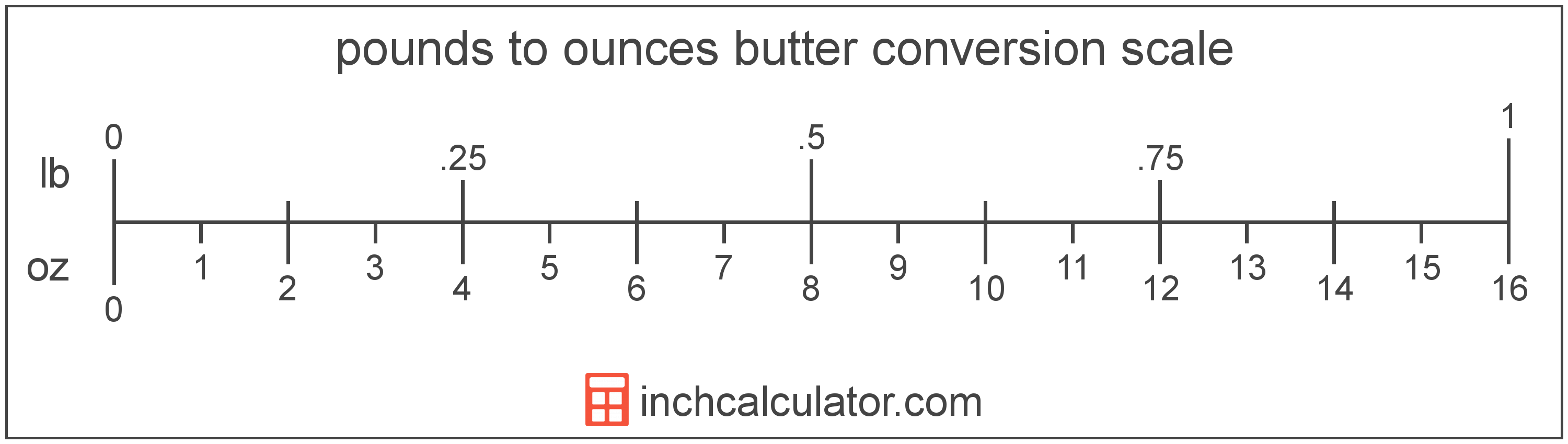# Convert Pounds of Butter to Ounces

Enter the butter in pounds below to get the value converted to ounces.

Results in Ounces:1 lb = 16 oz

Do you want to convert ounces to pounds?

## How to Convert Pounds to Ounces

To convert a measurement in pounds to a measurement in ounces, multiply the butter by the following conversion ratio: 16 ounces/pound.

Since one pound of butter is equal to 16 ounces, you can use this simple formula to convert:

ounces = pounds × 16

The butter in ounces is equal to the butter in pounds multiplied by 16.

For example, here's how to convert 5 pounds to ounces using the formula above.
ounces = (5 lb × 16) = 80 oz### How Many Ounces Are in a Pound of Butter?

There are 16 ounces in a pound of butter, which is why we use this value in the formula above.

1 lb = 16 oz

## What Is a Pound?

Butter in the US is most commonly sold by the pound, which contains 4 sticks, or 16 ounces.

The pound is a US customary and imperial unit of weight. Pounds can be abbreviated as lb; for example, 1 pound can be written as 1 lb.

## What Is an Ounce?

In the US butter is sold in 16 ounce packages, which contain 1 pound of butter.

The ounce is a US customary and imperial unit of weight. Ounces can be abbreviated as oz; for example, 1 ounce can be written as 1 oz.

## Pound to Ounce Conversion Table

Table showing various pound measurements converted to ounces.
Pounds Ounces
1 lb 16 oz
2 lb 32 oz
3 lb 48 oz
4 lb 64 oz
5 lb 80 oz
6 lb 96 oz
7 lb 112 oz
8 lb 128 oz
9 lb 144 oz
10 lb 160 oz
11 lb 176 oz
12 lb 192 oz
13 lb 208 oz
14 lb 224 oz
15 lb 240 oz
16 lb 256 oz
17 lb 272 oz
18 lb 288 oz
19 lb 304 oz
20 lb 320 oz
21 lb 336 oz
22 lb 352 oz
23 lb 368 oz
24 lb 384 oz
25 lb 400 oz
26 lb 416 oz
27 lb 432 oz
28 lb 448 oz
29 lb 464 oz
30 lb 480 oz
31 lb 496 oz
32 lb 512 oz
33 lb 528 oz
34 lb 544 oz
35 lb 560 oz
36 lb 576 oz
37 lb 592 oz
38 lb 608 oz
39 lb 624 oz
40 lb 640 oz